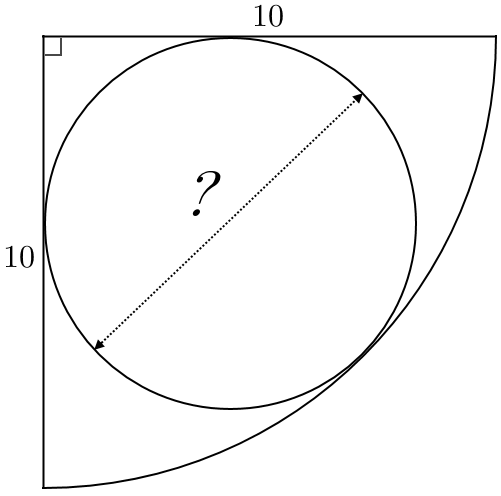# Circle-ception

Geometry Level 2A quadrant of a circle of radius $10$ has a circle inscribed in it - its diameter can be written as $a\left( \sqrt { b } -c \right)$ in fully factorised form, where $b$ is square free and $a$, $b$ and $c$ are integers.

Find the value of$\large\left\lfloor \frac { { a }^{ 2 }{ b }^{ 2 }{ c }^{ 2 } }{ a+b+c } \right\rfloor.$

×